# Nonlinear System Identification with NARMAX

How to identify a dynamical system from measurements.
Author

Murat Koptur

Published

September 7, 2022

# Introduction

System identification is a way to determine a system’s mathematical description by analyzing the system’s observed inputs and outputs. Definitely, the dynamics of the mathematical model that produced this signal from the measured input are hidden inside the output signal; how can this information be extracted? System identification provides a solution to this problem. Even under ideal conditions, this is difficult because the model of the system will be unknown, such as whether it is linear or nonlinear, how many terms are in the model, what type of terms should be in the model, whether the system has a time delay, what type of nonlinearity describes this system, and so on. Yet, if system is to be useful, these problems must be resolved. The benefits of system identification are numerous: it is applicable to all systems, it is frequently fast, and it can be used to track changes in the system$$^1$$.

What do we mean with “system”? One can think of “system” as any set of mathematical operations that takes one or more inputs and produces one or more outputs. Anything that can be related to input and output data is a system example: electrical systems, mechanical systems, biological systems, financial systems, chemical systems…$$^3$$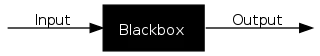Source: $$^2$$

Systems can be considered of two types, depending on whether they satisfy the principle of superposition:

• Linear systems are satisfies the superposition principle: if system is linear, then if input $$X_1$$ generates response $$Y_1$$ and input $$X_2$$ generates response $$Y_2$$, then input $$X_1 + X_2$$ generates response $$Y_1 + Y_2$$.
• On the other hand, nonlinear systems does not satisfy the superposition principle. This description is very vague, but there are so many types of nonlinear systems, it is almost impossible to write down a description that covers all the classes $$^1$$.

Most of real-world dynamical systems are nonlinear. In this article, we will not talk about linearization of nonlinear systems, as we will focus on the NARMAX method.

Following steps are needed for NARMAX modelling $$^3$$:

1. Collecting data;
2. Choice of mathematical representation;
3. Detecting model structure;
4. Parameter estimation;
5. Model validation;
6. Analysis of model.

NARMAX method was introduced in 1981 by Stephen A. Billings, NARMAX models are able to represent the most different and complex nonlinear systems. NARMAX models can be represented as:

$y_k= F^\ell[y_{k-1}, \dotsc, y_{k-n_y},x_{k-d}, x_{k-d-1}, \dotsc, x_{k-d-n_u}, e_{k-1}, \dotsc, e_{k-n_e}] + e_k$

where $$n_y\in \mathbb{N}$$, $$n_u \in \mathbb{N}$$ and $$n_e \in \mathbb{N}$$ are the maximum lags for the system output and system input and system noise , respectively; $$x_k \in \mathbb{R}^{n_x}$$ is the system input and $$y_k \in \mathbb{R}^{n_y}$$ is the system output at discrete time $$k \in \mathbb{N}^n$$; $$d$$ is the time delay; $$e_k \in \mathbb{R}^{n_e}$$ is system uncertainty and noise at discrete time $$k$$, (source: $$^3$$).

To approximate the unknown mapping $$f[\cdot]$$, we can use several nonlinear functions like $$^1$$:

• Polynomial basis: Polynomial NARMAX model can be written as

\begin{aligned} y(k)=\theta_0 &+\sum_{i_i=1}^n f_{i_1}\left(x_{i_1}(k)\right)+\sum_{i_1=1}^n \sum_{i_2=i_1}^n f_{i_1 i_2}\left(x_{i_1}(k), x_{i_2}(k)\right)+\cdots \\ &+\sum_{i_1=1}^n \cdots \sum_{i_{\ell}=i_{l-1}}^n f_{i_1 i_2 \cdots i_l}\left(x_{i_1}(k), x_{i_2}(k), \ldots, x_{i_{\ell}}(k)\right)+e(k) \end{aligned},

$f_{i_1 i_2 \cdots i_m}\left(x_{i_1}(k), x_{i_2}(k), \ldots, x_{i_m}(k)\right)=\theta_{i_1 i_2 \cdots i_m} \prod_{k=1}^m x_{i_k}(k), 1 \leq m \leq \ell,$

$x_m(k)= \begin{cases}y(k-m) & 1 \leq m \leq n_y \\ u\left(k-\left(m-n_y\right)\right) & n_y+1 \leq m \leq n_y+n_u \\ e\left(k-\left(m-n_y-n_u\right)\right) & n_y+n_u+1 \leq m \leq n_y+n_u+n_e\end{cases}$

where $$l$$ is the degree of polynomial nonlinearity, $$\theta_{i_1,i_2,\ldots,i_m}$$ are model parameters, and $$n=n_y+n_u+n_e$$, (source: $$^1$$).

Generalized additive models are defined as:

$y(k)=a_0+a_1y(k-1)+\cdots+a_{n_y}y(k-n_y)+b_1u(k-1)+\cdots+b_{n_u}u(k-n_u)+e(k)$

where $$y(k)$$, $$u(k)$$, $$e(k)$$, $$n_y$$, $$n_u$$ and $$n_e$$ are defined as before.

• Neural networks:

Recurrent NARX can be defined as:

$y(k)=F[\mathbf{x}(k)]=w_0+\sum_{i=1}^m w_i \phi_i(\mathbf{x}(k))+e(k)$

where $$\phi_i(\cdot)$$ is the nonlinear activation function.

RBF networks can be defined as:

$y(k)=F[\mathbf{x}(k)]=\sum_{i=1}^N w_i \phi(\|\mathbf{x}(k)-\mathbf{x}(i)\|)$

where $$\mathbf{x}(k)=[x_1(k),\ldots,x_n(k)]^T$$ is the $$k$$th observation vector ($$k=1,2,\ldots,N$$), $$\phi(||\mathbf{x}(k)-\mathbf{x}(i)||$$ are some arbitrary nonlinear functions known as radial basis functions or kernels, and $$W_i$$ are the unknown weights.

• Wavelet basis:

Wavelet NARMAX model can be defined as:

$y(k)=F^{(P)}[\mathbf{x}(k)]+F^{(W)}[\mathbf{x}(k)]+F^{(Z)}[\mathbf{z}(k)]+e(k)$

where $$F^{(P)}[\mathbf{x}(k)]$$ is polynomial model that is used to model any slow or smooth varying trends, F^{(W)}[(k)] is a wavelet model that is used to model rapid changes or nonsmooth dynamics, and F^{(Z)}[(k)] is a linear or nonlinear moving average model that models the noise:

$F^{(W)}[\mathbf{x}(k)] = c_0+F_1[\mathbf{x}(k)]+F_2[\mathbf{x}(k)]+\cdots+F_n[\mathbf{x}(k)]$

$\begin{gathered} F_1(\mathbf{x}(k))=\sum_{i=1}^n f_i\left(x_i(k)\right) \\ F_2(\mathbf{x}(k))=\sum_{i=1}^n \sum_{j=i+1}^n f_{i j}\left(x_i(k), x_j(k)\right) \\ F_m(\mathbf{x}(k))=\sum_{1 \leq i_1<i_2<\cdots<i_m \leq n} f_{i_1 i_2 \cdots i_m}\left(x_{i_1}(k), x_{i_2}(k), \ldots, x_{i_m}(k)\right), 2<m<n \\ F_n(\mathbf{x}(k))=f_{12 \cdots n}\left(x_1(k), x_2(k), \ldots, x_n(k)\right) \end{gathered}$

where $$c_0$$ is constant and $$F_i[\cdot]$$ are individual wavelet sub-models.

# Examples

## Lorenz system

The Lorenz system is a system of ordinary differential equations defined as:

\begin{align*} \frac{dx}{dt} &= \sigma(y-x) \\ \frac{dy}{dt} &= x(\rho-z)-y\\ \frac{dz}{dt} &= xy - \beta z \end{align*} where $$\sigma, \rho, \beta$$ are model parameters.

Let’s simulate the data with $$\rho=28, \sigma=10, \beta=8/3$$. Here is the time step $$dt$$ is $$0.01$$, final time $$10$$, initial values $$(x_0,y_0,z_0)$$ is $$(0.1, 0.1, 0.1)$$:

rho = 28
sigma = 10
beta = 8/3
dt = 0.01
T = 10
n = int(T / dt)
t = np.linspace(0, T, n)

x = np.zeros(n)
y = np.zeros(n)
z = np.zeros(n)

x = 0.1
y = 0.1
z = 0.1

for i in range(n - 1):
x[i + 1] = x[i] + dt * (sigma * (y[i] - x[i]))
y[i + 1] = y[i] + dt * (x[i] * (rho - z[i]) - y[i])
z[i + 1] = z[i] + dt * (x[i] * y[i] - beta * z[i] )

fig, ax = plt.subplots(3, 1)
ax.plot(t, x, lw=2)
ax.plot(t, y, lw=2)
ax.plot(t, z, lw=2)

ax.set_title("x", loc="right")
ax.set_title("y", loc="right")
ax.set_title("z", loc="right")
Text(1.0, 1.0, 'z')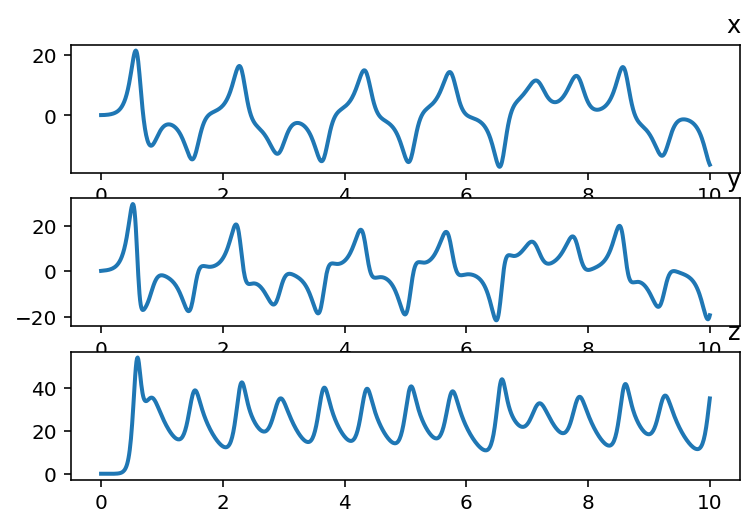px.line_3d(x=z,y=y,z=x, title="Lorenz system") # xz axis inverted for sake of plot

Divide data to train and validation sets, last 50 observations will be validation set:

test_size = 50

x_train, x_valid = temporal_train_test_split(pd.Series(x), test_size=test_size)
y_train, y_valid = temporal_train_test_split(pd.Series(y), test_size=test_size)
z_train, z_valid = temporal_train_test_split(pd.Series(z), test_size=test_size)

plot_series(x_train, x_valid, labels=["x_train", "x_valid"])
plot_series(y_train, y_valid, labels=["y_train", "y_valid"])
plot_series(z_train, z_valid, labels=["z_train", "z_valid"])
(<Figure size 1152x288 with 1 Axes>, <AxesSubplot:>)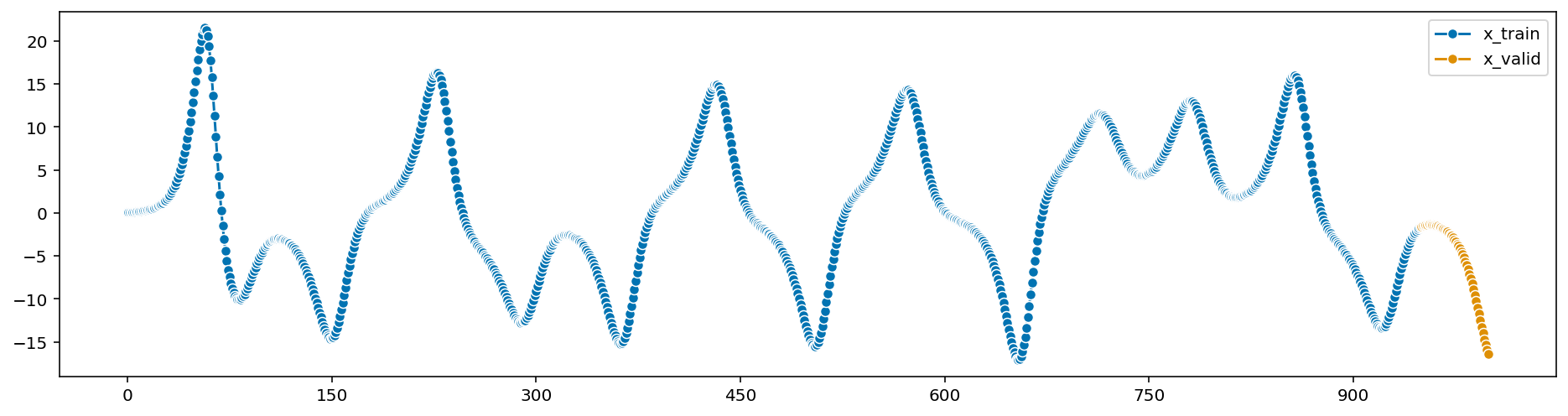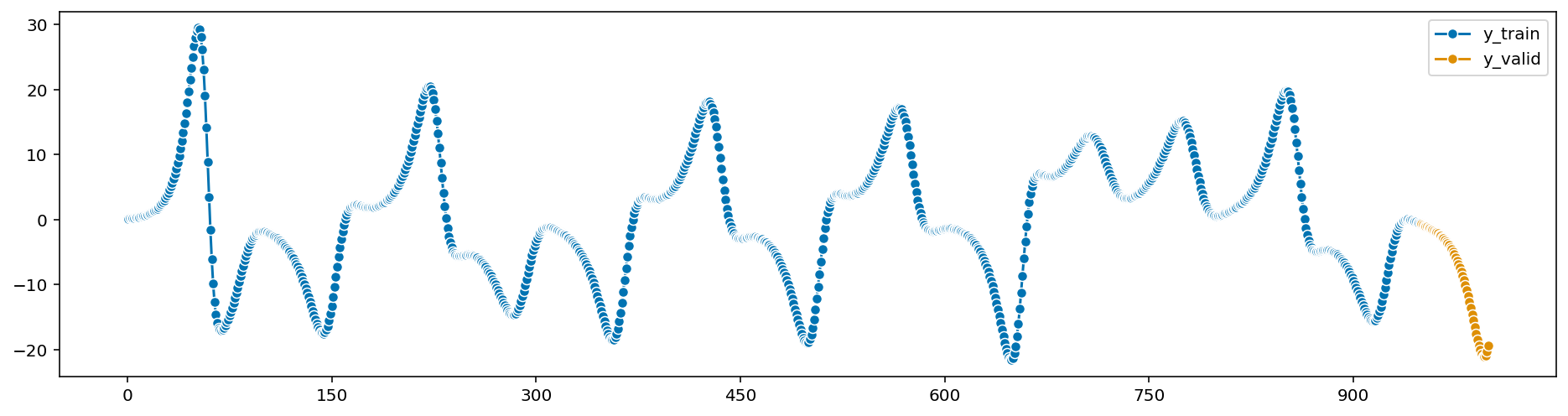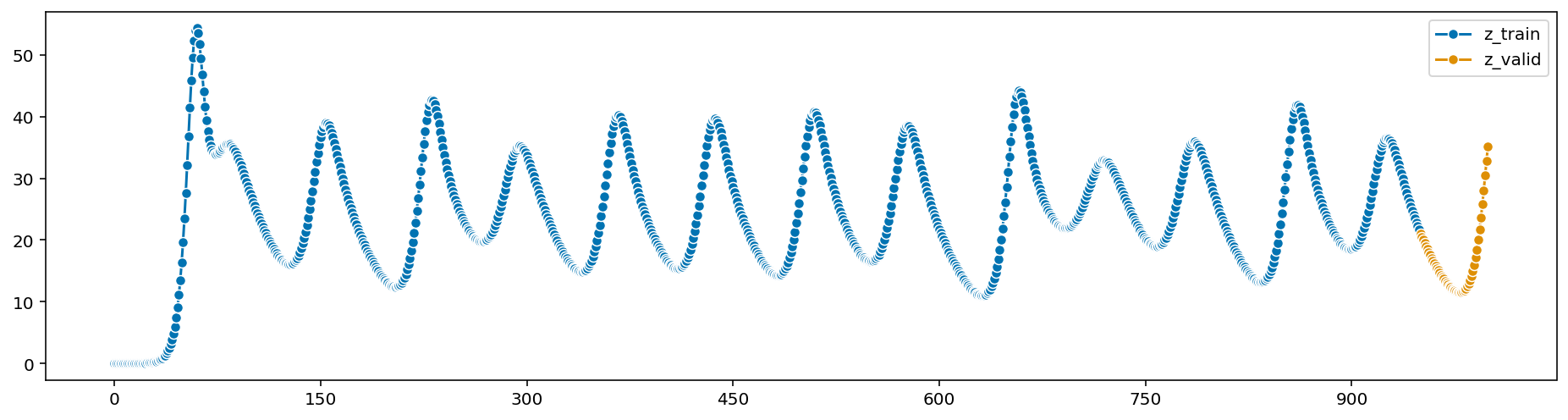Computations:

from sysidentpy.model_structure_selection import FROLS
from sysidentpy.basis_function._basis_function import Polynomial, Fourier
from sysidentpy.utils.display_results import results
from sysidentpy.utils.plotting import plot_residues_correlation, plot_results
from sysidentpy.residues.residues_correlation import compute_residues_autocorrelation, compute_cross_correlation

# Reshape data (needed for sysidentpy)
x_train = x_train.values.reshape(-1, 1)
x_valid = x_valid.values.reshape(-1, 1)
y_train = y_train.values.reshape(-1, 1)
y_valid = y_valid.values.reshape(-1, 1)
z_train = z_train.values.reshape(-1, 1)
z_valid = z_valid.values.reshape(-1, 1)

# Our polynomial basis functions with max degrees 5
basis_function = Polynomial(degree=5)

# Forward Regression with Orthogonal Least Squares (FOLRS) model structure identification
modelx = FROLS(
order_selection=True,
ylag=4,
elag=4,
info_criteria='aic',
estimator='recursive_least_squares',
basis_function=basis_function,
model_type='NAR' # we don't have exogenous variables
)

# Fit model to training data
modelx.fit(y=x_train)

# Add needed lags to validation data
x_valid = np.concatenate([x_train[-modelx.max_lag:], x_valid])

# Predict the validation data
xhat = modelx.predict(y=x_valid, forecast_horizon=test_size)

Calculate MSE error score:

from sktime.performance_metrics.forecasting import mean_squared_error

modelx_loss = mean_squared_error(x_valid, xhat)
print(modelx_loss)
0.0033133566986800886

Regression coefficients:

r = pd.DataFrame(
results(
modelx.final_model, modelx.theta, modelx.err,
modelx.n_terms, err_precision=8, dtype='sci'
),
columns=['Regressors', 'Parameters', 'ERR'])
print(r)
           Regressors   Parameters             ERR
0              y(k-1)   3.7758E+00  9.95972552E-01
1              y(k-2)  -5.3235E+00  3.97965581E-03
2              y(k-3)   3.3225E+00  4.63994821E-05
3              y(k-4)  -7.7480E-01  1.31535686E-06
4            y(k-1)^3  -1.3230E-05  1.32545331E-08
5      y(k-3)^2y(k-2)   3.5535E-04  6.13642972E-08
6            y(k-4)^5   9.6040E-09  6.44836942E-10
7  y(k-4)y(k-3)y(k-1)   4.3154E-04  2.56203519E-10
8      y(k-4)y(k-2)^2  -7.7419E-04  8.56524112E-10

Plot prediction results:

plot_results(y=x_valid[modelx.max_lag:], yhat=xhat[modelx.max_lag:])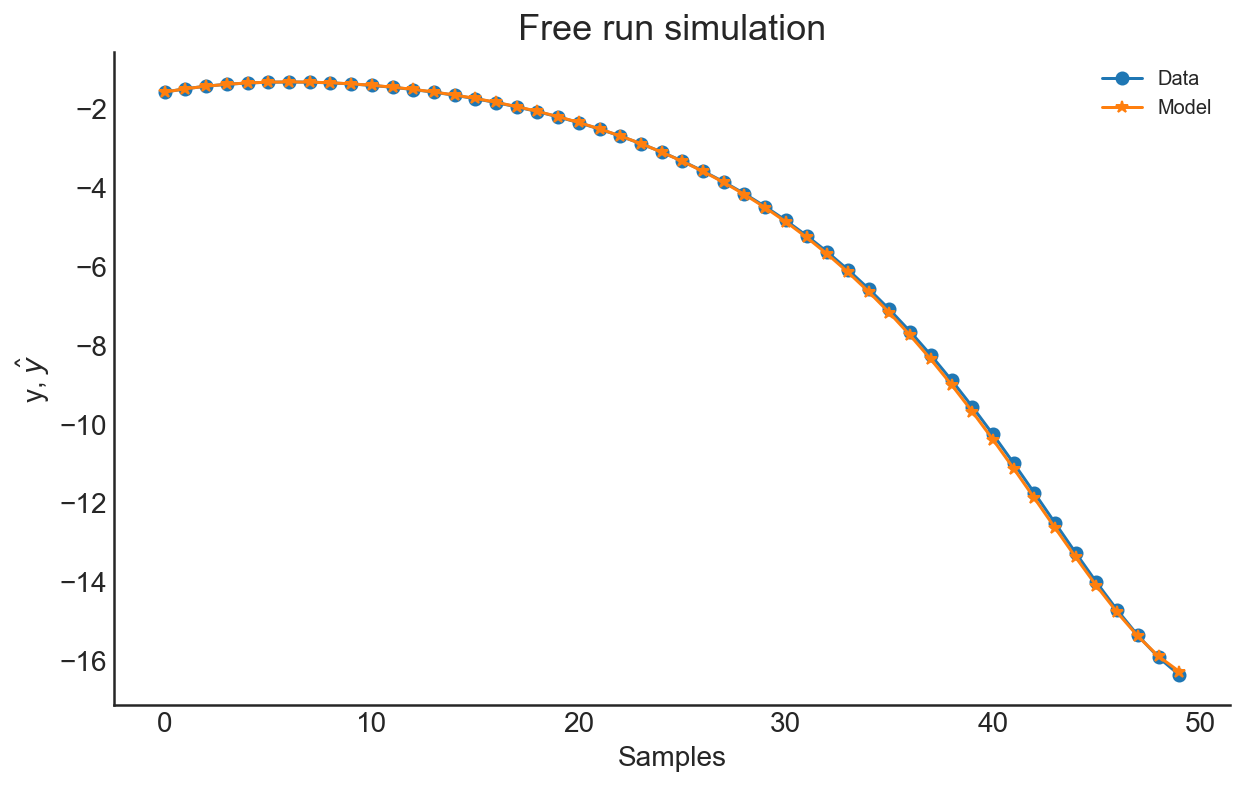We’ve a perfect fit.

Residual autocorrelation and cross-correlations:

ee = compute_residues_autocorrelation(x_valid, xhat)
plot_residues_correlation(data=ee, title="Residues", ylabel="$e^2$")
x1e = compute_cross_correlation(x_valid, xhat, x_valid)
plot_residues_correlation(data=x1e, title="Residues", ylabel="$x_1e$")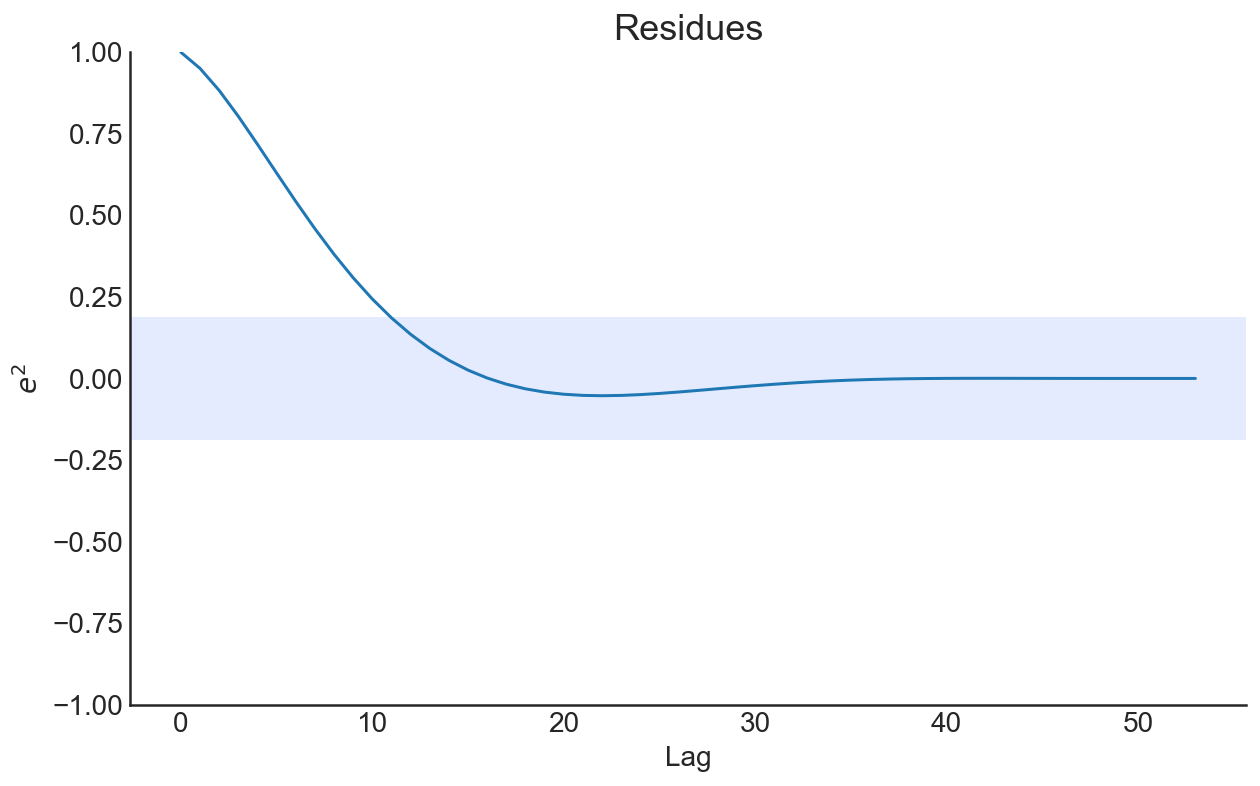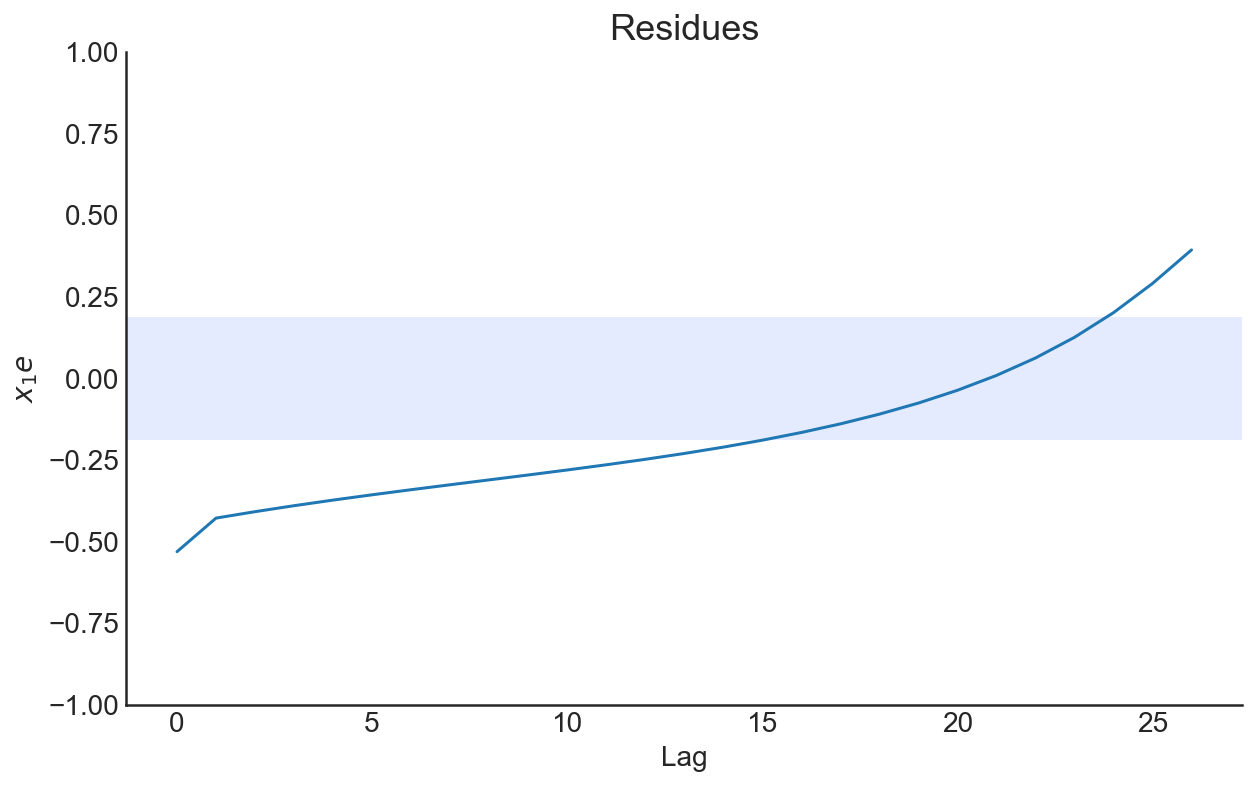$$y$$ and $$z$$ components of the Lorenz model also can be estimated above. Now, let’s add measurement noise to our data and try to estimate it.

## Noisy Lorenz System

Now we will assume that we observed the data with independent and identically distributed measurement noise (we will assume that measurement noise is distributed as uniform on interval [-1, 1]):

$\text{Observed Data} = \text{Process Outcome} + \text{Measurement Noise}$

Simulate the noisy Lorenz system:

# ...
# add measurement noise to observations
x = x + np.random.uniform(-1,1,n)
y = y + np.random.uniform(-1,1,n)
z = z + np.random.uniform(-1,1,n)
fig, ax = plt.subplots(3, 1)
ax.plot(t, x, lw=2)
ax.plot(t, y, lw=2)
ax.plot(t, z, lw=2)

ax.set_title("x", loc="right")
ax.set_title("y", loc="right")
ax.set_title("z", loc="right")

px.line_3d(x=z,y=y,z=x, title="Noisy Lorenz system")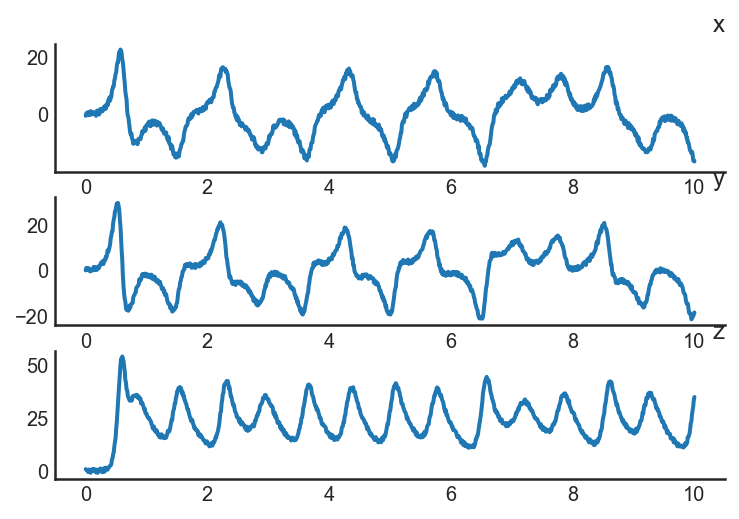Now apply same method and look results:

Loss:

modelx_loss = mean_squared_error(x_valid, xhat)
print(modelx_loss)
3.1303289624476904

Regression coefficients:

r = pd.DataFrame(
results(
modelx.final_model, modelx.theta, modelx.err,
modelx.n_terms, err_precision=8, dtype='sci'
),
columns=['Regressors', 'Parameters', 'ERR'])
print(r)
                Regressors   Parameters             ERR
0                   y(k-1)   4.5142E-01  9.86393489E-01
1          y(k-11)^2y(k-7)  -9.1477E-04  2.95518502E-03
2                   y(k-2)   3.0778E-01  1.52411152E-03
3        y(k-11)^3y(k-9)^2   1.4444E-06  5.72445300E-04
4                   y(k-3)   3.6355E-01  3.32725057E-04
5                   y(k-9)  -1.5725E-01  3.16618507E-04
6  y(k-10)^2y(k-3)y(k-2)^2  -6.5121E-07  1.64874661E-04
7                   y(k-4)   1.0455E-01  1.71874614E-04

Plot prediction results:

plot_results(y=x_valid[modelx.max_lag:], yhat=xhat[modelx.max_lag:])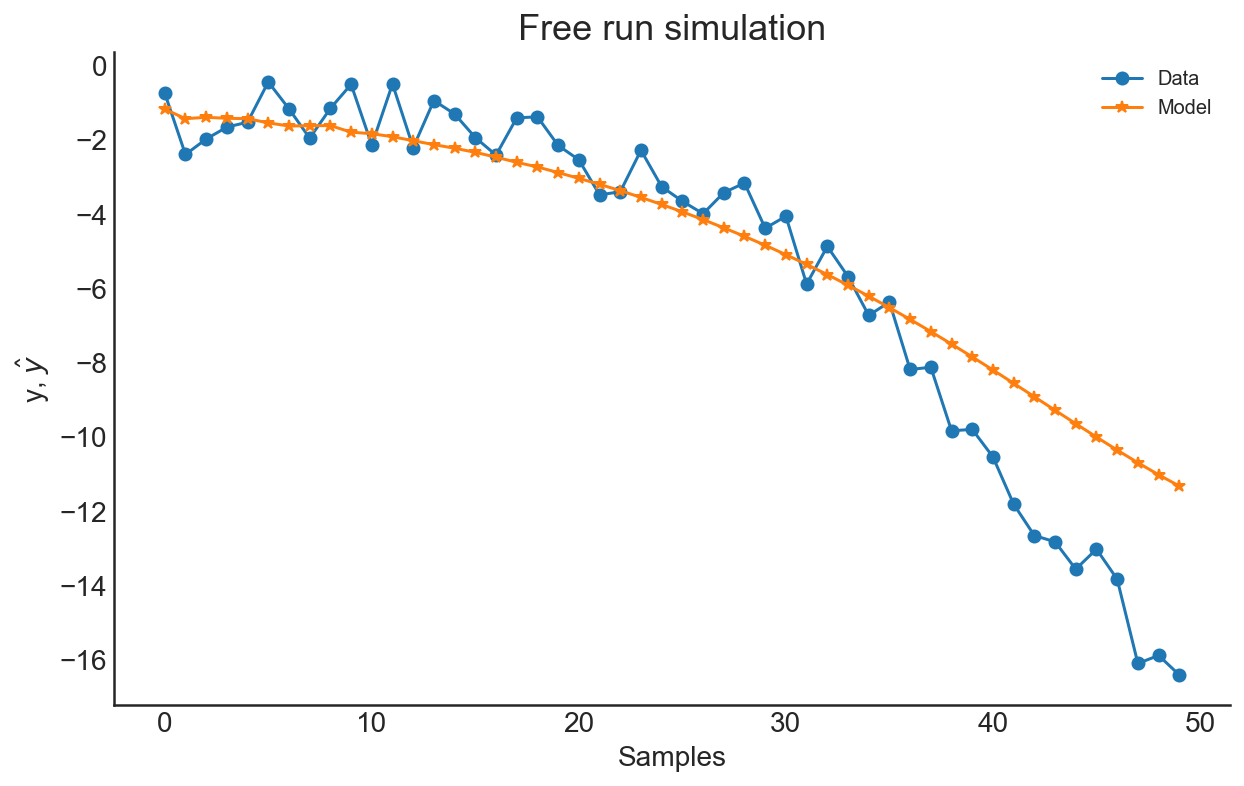In the presence of noise, we added more lagged variables to the model and we tried to approximate the nature of the process.

Residual autocorrelation and cross-correlations:

ee = compute_residues_autocorrelation(x_valid, xhat)
plot_residues_correlation(data=ee, title="Residues", ylabel="$e^2$")
x1e = compute_cross_correlation(x_valid, xhat, x_valid)
plot_residues_correlation(data=x1e, title="Residues", ylabel="$x_1e$")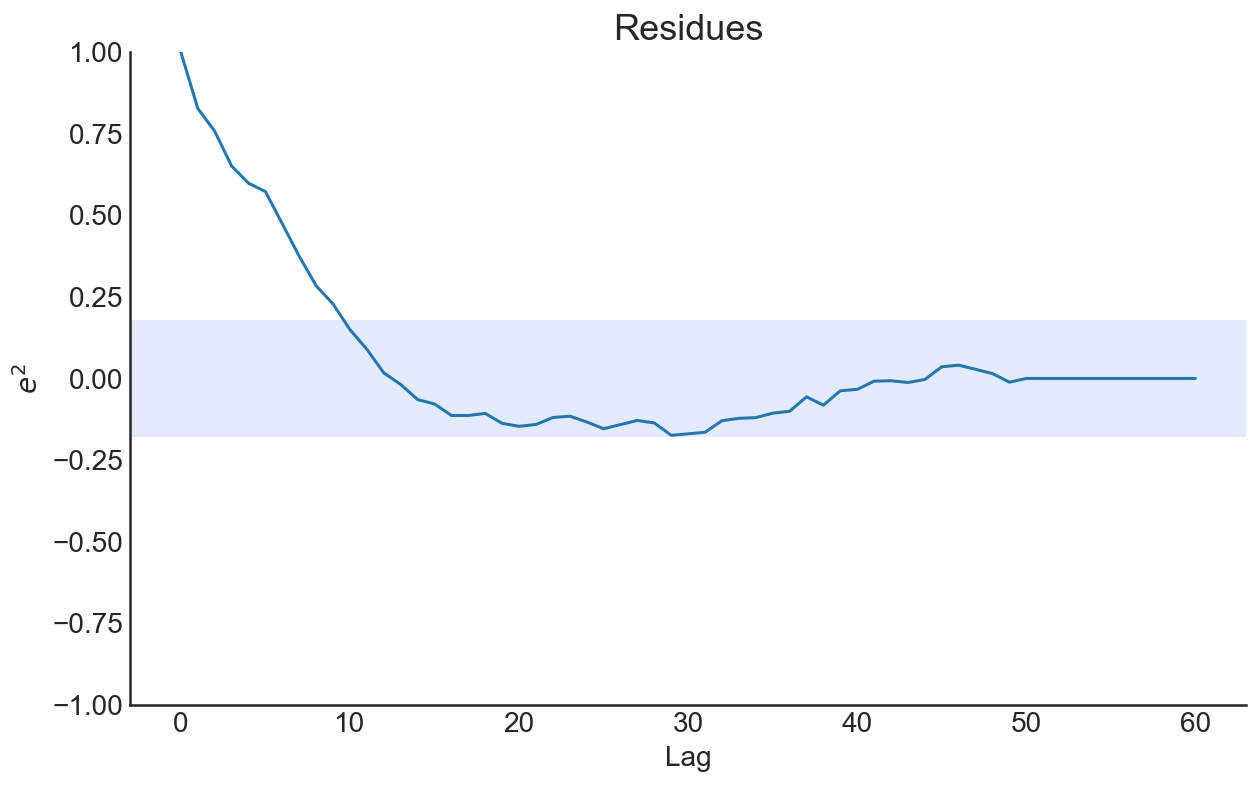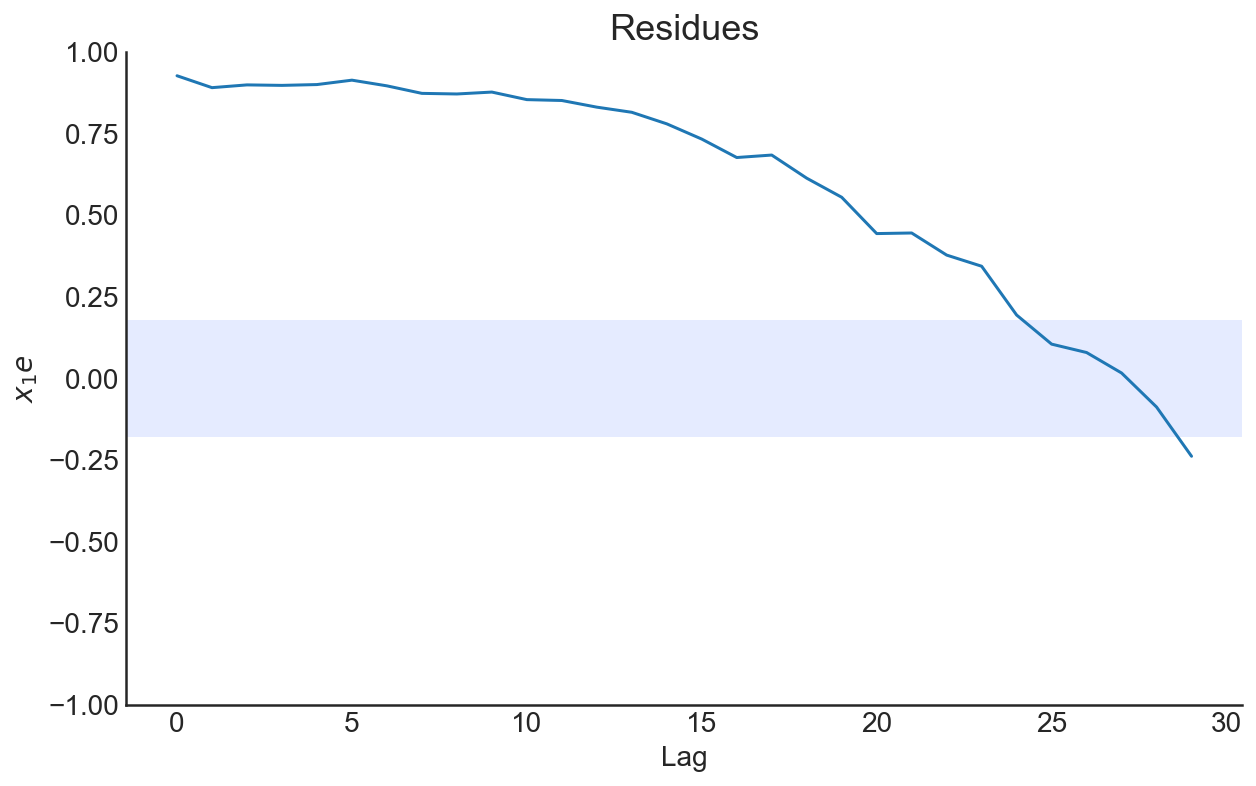Full source code: https://github.com/mrtkp9993/MyDsProjects/tree/main/SysIdent

## Citation

BibTeX citation:
@online{koptur2022,
author = {Murat Koptur},
title = {Nonlinear {System} {Identification} with {NARMAX}},
date = {2022-09-07},
url = {https://www.muratkoptur.com/MyDsProjects/Analysis.html},
langid = {en}
}